# Reciprocal of Menelaus' Theorem

## Famous theorems in 2D Geometry

Reciprocal of Menelaus' theorem. Whether triangle ABC and the points M, N, P located on the lines BC, CA, respectively, AB different peaks A, B, C. If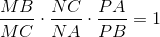, the points M, N, P are collinear.

Proof. We have this figure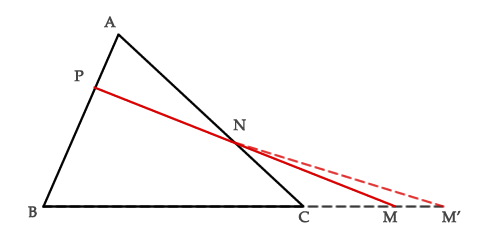We suppose, hypothetically, that the points M, N, P are not collinear. Then there is the point M'∈ BC, so that M', N, P are collinear.
And then there is the relationship (according  Menelaus's Theorem):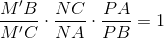But we already have:Further, we obtain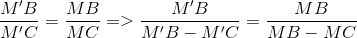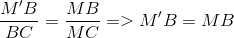So though, we have M' = M. And, at the end, M, N, P  are collinear.

Keywords: geometry, Menelaus, reciprocal, mutual, triangle, collinear

### Forum

Here you can discuss about mathematic, about algebra, geometry, trigonometry.

It is not mandatory to be logged in on this forum but it is nice to have an account. You can ask about mathematics just with your name and your email.

This maths forum is one of the easiest forums to use it.

>> Go to Math Forum